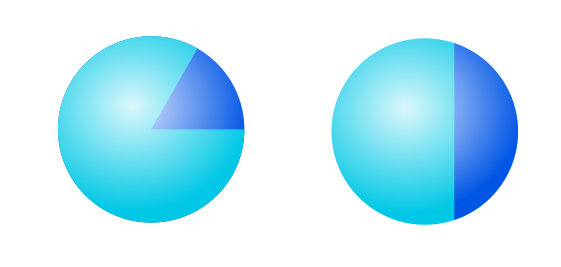# How to create dual-colored sphere

Hello all, I’d like to create partially colored spheres which always face the camera. Partially colored means exactly 2 colors, and the fraction of 1 color to another varies based on the context. The sphere can be colored in the sense of a “pie chart” or more simply like in my example drawing.

The context may help in understanding the problem. The sphere represents an atomic position which can be occupied by one of two different elemental species, with one of these species possibly more likely than the other. The two colors represent the two separate species, and the “amount” of one color to the other represents the probability of one element occupying the position.

I’m not sure how to approach programmatically coloring the spheres in this manner, but keeping them facing the camera should be simple using vtkFollower.Something like this:

``````from vedo import *
def atom(pos, f):
s = Sphere(res=(360,20), r=0.5)
s.lighting("glossy").follow_camera()
pts = s.cell_centers()
angle = 180-np.arctan2(pts[:,1], pts[:,0])*57.3
ids = np.where(angle<f)
cols = np.zeros([s.ncells,3])+[200,200,255]
cols[ids] = [10,10,255]
s.cellcolors = cols
return s.pos(pos)
show(
atom([0,0,0], 30),
atom([3,2,1], 70),
atom([2,3,2], 120),
axes=1,
)
``````